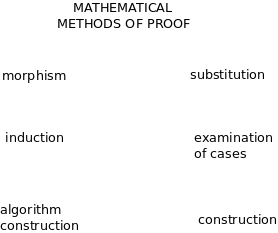# Book: MethodsOfProof

See: Math concepts

Make sense, if possible, of the six methods of mathematical proof.

• Study the proofs of famous theorems.
• How are the six methods of proof related to the ways of figuring things out? Study examples of proofs and of problem solving.
• Why are there so many ways of solving the Pythagorean theorem?Mathematical induction can be thought of as a proof by contradiction where we have organized the conditions in an infinite sequence and we are looking at the first one to fail and proving that it doesn't fail.

Parsiųstas iš http://www.ms.lt/sodas/Book/MethodsOfProof
Puslapis paskutinį kartą pakeistas 2018 lapkričio 11 d., 16:29